Next: Experimental Details Up: SR Spectroscopy Previous: Fast Fourier Transform (FFT)   Contents

# Zero-Field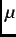SR (ZF-SR)

One of the advantages ofSR spectroscopy over other magnetic resonance techniques (i.e. NMR, ESR) is that measurements can be performed in zero external magnetic field. In a zero-field muon-spin relaxation (ZF-SR) experiment (Fig. 2.4), muons are used to probe the local magnetic field at their stopping site due to nearby nuclear and/or electronic magnetic moments. This is achieved by measuring the time evolution of the muon-spin polarization along its original direction (i.e. the relaxation function).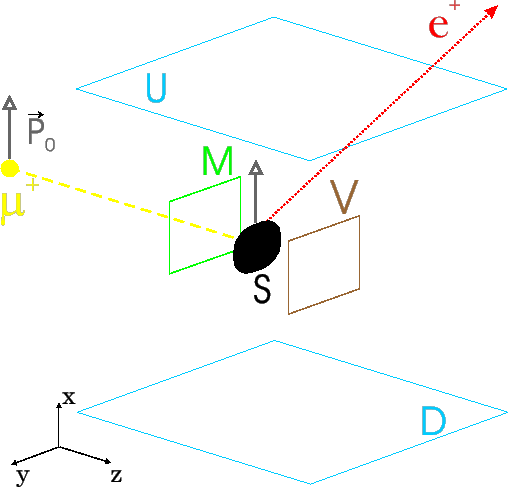If the local magnetic fields are static, the muon will Larmor precess about the component of local field that is perpendicular to the muon spin. For the case of a sample with magnetic order, this gives rise to a muon-spin precession signal with a discrete frequency oscillation for each magnetically inequivalent muon site in the crystallographic lattice. The observed ZF relaxation function is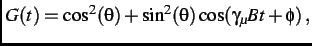(3.11)

where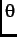is the angle between the muon spin and the average static internal field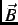.

The magnetic field distribution sensed by the muons in a system consisting of dense randomly oriented static magnetic moments is a Gaussian function. The corresponding relaxation function is given by the famous (Gaussian) Kubo-Toyabe (KT) function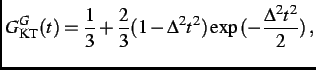(3.12)

where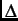is the relaxation rate and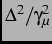is the width of the magnetic field distribution at the muon site. Note that for early times (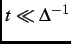) or for slow relaxation, Eq. (2.12) can be Taylor expanded as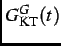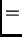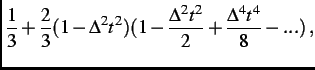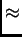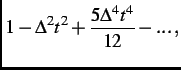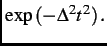(3.13)

The Gaussian KT function is routinely used to describe nuclear magnetic moments, which are static on theSR time scale.

A Lorentzian magnetic field distribution is sensed by the muons in a system consisting of dilute randomly oriented static magnetic moments (i.e. moments that are distributed randomly on less than 1% of the lattice sites). The corresponding relaxation function is a Lorentzian KT function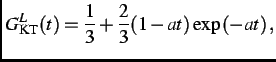(3.14)

whereis the relaxation rate. For early times (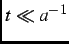) or for slow relaxation, Eq. (2.14) can be Taylor expanded as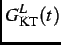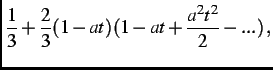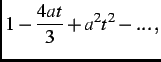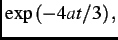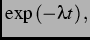(3.15)

where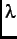=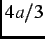is the relaxation rate. The above KT functions describe dense/dilute randomly oriented static magnetic moments. For the situation where the local magnetism is intermediate between the dilute and dense limits, or dilute electronic magnetic moments (or magnetic clusters) are embedded in a system of concentrated nuclear dipoles, some combination of Eq. (2.13) and Eq. (2.15) is required.

If the magnetic moments in the sample are slowly fluctuating i.e.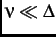where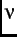is the fluctuation rate, the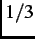tail'' in the KT functions relax to zero. If the magnetic moments are fluctuating at a high rate such that>, the relaxation function then becomes an exponential function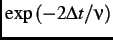. For intermediate values of, the relaxation function has no analytical form.Next: Experimental Details Up: SR Spectroscopy Previous: Fast Fourier Transform (FFT)   Contents
Jess H. Brewer 2003-07-01